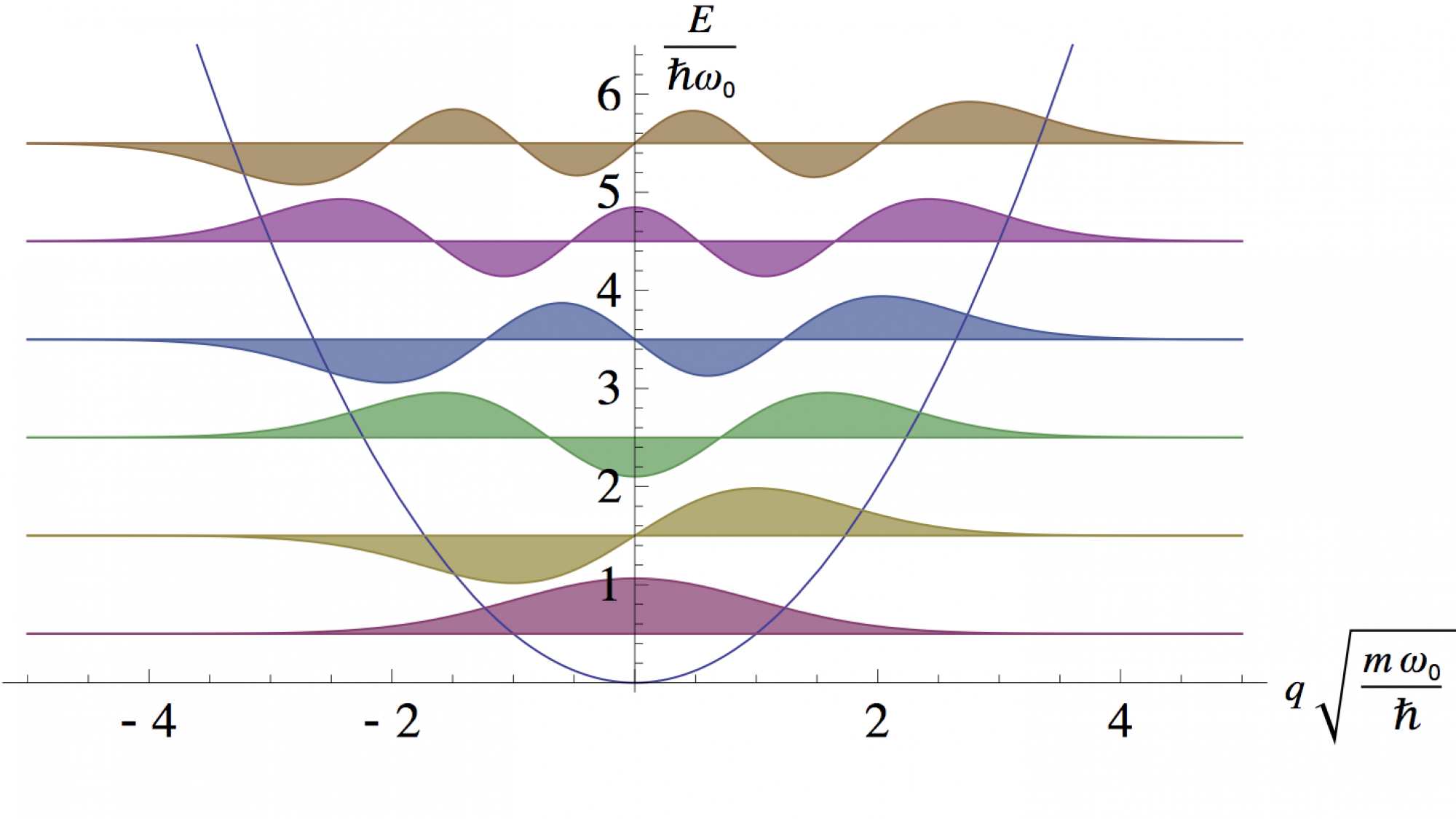# Ab initio modeling | Theoretical physics

Our research is focused on theoretical and computational condensed matter physics.
By utilizing theoretical and computational approaches our group studies mesoscopic systems, such as superconducting qubits, and normal and superfluid properties of a Fermi liquid applied to liquid Helium-3. For more information, contact professor Matti Alatalo (matti.alatalo(at)oulu.fi) or PI Matti Silveri (matti.silveri(at)oulu.fi).040 7541759

## Research group description

By utilizing theoretical and computational approaches our group studies mesoscopic systems, such as superconducting qubits, and normal and superfluid properties of a Fermi liquid applied to liquid Helium-3. For more information, contact professor Matti Alatalo (matti.alatalo(at)oulu.fi) or PI Matti Silveri (matti.silveri(at)oulu.fi).

The main purpose of theoretical physics is to understand physical phenomena. Accurate understanding is possible only using mathematical models. In the best case, experimental observations can be explained using only a small number of basic assumptions. Using the models it is possible to calculate the properties of the system under different conditions and predict new phenomena.The figure shows different length scales in the universe. Every division in the scale corresponds to a length scale that is ten times larger than the one below. The length scales from the atomic size to the human size are shown as enlarged on the right hand side.

Atoms and its constituent particles are described by laws of quantum mechanics. On the human scale these phenomena are averaged, and the averages obey laws of classical physics. Between these scales there is a mesoscopic region, where the behavior is partly classical but partly quantum mechanical.

Research of theoretical physics in Oulu concentrates on the scale from atomic to mesoscopic physics. This range shows many interesting phenomena, and some examples are given below.